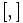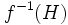# 2-subnormal subgroup

## Definition

QUICK PHRASES: normal inside normal closure, every conjugate is in its normalizer, normal closure is in normalizer, normal subgroup of normal subgroup, subgroup of subnormal defect at most two
No. Shorthand A subgroup of a group is 2-subnormal in it if ... A subgroup$H$ of a group$G$ if 2-subnormal in$G$ if ...
1 Normal of normal there is an intermediate subgroup containing it such that the subgroup is normal in the intermediate subgroup and such that the intermediate subgroup is normal in the whole group. there is subgroup$K$ of$G$ such that$H$ is a normal subgroup of$K$ and$K$ is a normal subgroup of$G$.
2 Normal in closure the subgroup is normal in its normal closure in the whole group.$H$ is a normal subgroup of its normal closure$H^G$ in$G$.
3 Normal closure in normalizer the normal closure of the subgroup is contained in the normalizer of the subgroup. the normal closure$H^G$ is contained in the normalizer$N_G(H)$.
4 Every conjugate in normalizer every conjugate of the subgroup is contained in its normalizer, i.e., every conjugate normalizes it. for every$g \in G$,$gHg^{-1} \le N_G(H)$.
5 In normal core of normalizer the subgroup is contained in its normal core of normalizer: the normal core of its normalizer.$H$ is contained in the normal core$(N_G(H))_G$ of$N_G(H)$ in$G$.
6 Subnormal of depth 2 it is a subnormal subgroup whose subnormal depth (also called subnormal defect) is at most$2$.$H$ is subnormal in$G$ with subnormal depth at most$2$.
7 contains second commutator it contains its second commutator subgroup with the whole group$[[G,H],H] \le H$ where$[,]$ denotes the commutator of two subgroups.
This definition is presented using a tabular format. |View all pages with definitions in tabular format
VIEW RELATED: Analogues of this | Variations of this | Opposites of this |
View a complete list of semi-basic definitions on this wiki
This page describes a subgroup property obtained as a composition of two fundamental subgroup properties: normal subgroup and normal subgroup
View other such compositions|View all subgroup properties
This is a variation of normality|Find other variations of normality | Read a survey article on varying normality

## Comment

A 2-subnormal subgroup$H$ has a unique fastest ascending subnormal series$H \le K \le G$, where$K$ is the normal core of$N_G(H)$. It also has a unique fastest descending subnormal series$G \ge L \ge H$, where$L$ is the normal closure of$H$ in$G$. While subnormal subgroups of larger depth also have unique fastest descending subnormal series, they do not in general possess unique fastest ascending subnormal series. Further information: 2-subnormal subgroup has a unique fastest ascending subnormal series, Subnormal subgroup has a unique fastest descending subnormal series, 3-subnormal subgroup need not have a unique fastest ascending subnormal series

## Formalisms

### First-order description

This subgroup property is a first-order subgroup property, viz., it has a first-order description in the theory of groups.
View a complete list of first-order subgroup properties

A subgroup$H$ is 2-subnormal in a group$G$ if it satisfies the following first-order sentence:$\forall g \in G, \forall x,y \in H, gxg^{-1}ygx^{-1}g^{-1} \in H$

## Examples

VIEW: subgroups of groups satisfying this property | subgroups of groups dissatisfying this property
VIEW: Related subgroup property satisfactions | Related subgroup property dissatisfactions

## Relation with other properties

### Stronger properties

Property Meaning Proof of implication Proof of strictness (reverse implication failure) Intermediate notions
Base of a wreath product occurs as the base (the direct factor in the direct product that forms the normal subgroup of the semidirect product) of a wreath product Base of a wreath product with diagonal action, Central factor of normal subgroup, Direct factor of complemented normal subgroup, Direct factor of normal subgroup, Right-transitively 2-subnormal subgroup, Transitively normal subgroup of normal subgroup|FULL LIST, MORE INFO
Normal subgroup invariant under inner automorphisms; subnormal of depth at most 1 (by definition) normality is not transitive 2-hypernormalized subgroup, Central factor of normal subgroup, Direct factor of normal subgroup, Join-transitively 2-subnormal subgroup, Modular 2-subnormal subgroup, Normal subgroup of characteristic subgroup, Permutable 2-subnormal subgroup, Transitively normal subgroup of normal subgroup|FULL LIST, MORE INFO
2-hypernormalized subgroup normalizer is normal 2-subnormal not implies hypernormalized |FULL LIST, MORE INFO
Right-transitively 2-subnormal subgroup every 2-subnormal subgroup of it is 2-subnormal in the whole group. (by definition, since 2-subnormality is an identity-true subgroup property) 2-subnormality is not transitive |FULL LIST, MORE INFO
Left-transitively 2-subnormal subgroup If whole group is 2-subnormal in some group, so is subgroup (by definition, since 2-subnormality is an identity-true subgroup property) 2-subnormality is not transitive Normal subgroup of characteristic subgroup|FULL LIST, MORE INFO
Join-transitively 2-subnormal subgroup join with any 2-subnormal subgroup is 2-subnormal (by definition, since 2-subnormality is a trivially true subgroup property) 2-subnormality is not finite-join-closed |FULL LIST, MORE INFO
Commutator of a normal subgroup and a subset Commutator of a normal subgroup and a subset implies 2-subnormal |FULL LIST, MORE INFO
Direct factor of characteristic subgroup direct factor of characteristic subgroup Central factor of normal subgroup, Direct factor of normal subgroup, Normal subgroup of characteristic subgroup|FULL LIST, MORE INFO
Direct factor of normal subgroup direct factor of a normal subgroup of the whole group Central factor of normal subgroup, Transitively normal subgroup of normal subgroup|FULL LIST, MORE INFO
Normal subgroup of characteristic subgroup normal subgroup of a characteristic subgroup of the whole group follows from characteristic implies normal |FULL LIST, MORE INFO

### Weaker properties

Property Meaning Proof of implication Proof of strictness (reverse implication failure) Intermediate notions
Conjugate-permutable subgroup permutes with all conjugate subgroups 2-subnormal implies conjugate-permutable conjugate-permutable not implies 2-subnormal Permutable subgroup of normal subgroup|FULL LIST, MORE INFO
Join-transitively subnormal subgroup join with any subnormal subgroup is subnormal 2-subnormal implies join-transitively subnormal join-transitively subnormal not implies 2-subnormal Conjugate-join-closed subnormal subgroup, Intermediately join-transitively subnormal subgroup, Join of finitely many 2-subnormal subgroups, Linear-bound join-transitively subnormal subgroup, Polynomial-bound join-transitively subnormal subgroup|FULL LIST, MORE INFO
Join of finitely many 2-subnormal subgroups 2-subnormality is not finite-join-closed |FULL LIST, MORE INFO
Subnormal subgroup there exist subgroups of arbitrarily large subnormal depth 3-subnormal subgroup, 4-subnormal subgroup, Finite-conjugate-join-closed subnormal subgroup, Intermediately join-transitively subnormal subgroup, Join of finitely many 2-subnormal subgroups, Join-transitively subnormal subgroup, Linear-bound join-transitively subnormal subgroup|FULL LIST, MORE INFO
3-subnormal subgroup subnormal of depth at most 3 |FULL LIST, MORE INFO
4-subnormal subgroup subnormal of depth at most 4 |FULL LIST, MORE INFO

## Metaproperties

Metaproperty name Satisfied? Proof Statement with symbols
transitive subgroup property No 2-subnormality is not transitive There exist groups$H \le K \le G$, with$H$ 2-subnormal in$K$,$K$ 2-subnormal in$G$, but$H$ not 2-subnormal in$G$.
trim subgroup property Yes Every group is normal in itself, trivial subgroup is normal For any group$G$, the whole group and the trivial subgroup are both 2-subnormal.
strongly intersection-closed subgroup property Yes Subnormality of fixed depth is strongly intersection-closed$H_i, i \in I$ all 2-subnormal subgroups of$G$, then so is$\bigcap_{i \in I} H_i$.
finite-join-closed subgroup property No 2-subnormality is not finite-join-closed Can have subgroups$H, K \le G$, both 2-subnormal in$G$, such that$\langle H, K \rangle$ is not 2-subnormal.
conjugate-join-closed subgroup property Yes 2-subnormality is conjugate-join-closed A join of subgroups$H_i, i \in I$ of$G$, with all 2-subnormal in$G$ and all conjugate to each other, is also 2-subnormal.
intermediate subgroup condition Yes 2-subnormality satisfies intermediate subgroup condition If$H \le K \le G$, with$H$ 2-subnormal in$G$, then$H$ is 2-subnormal in$K$.
transfer condition Yes 2-subnormality satisfies transfer condition If$H, K \le G$, with$H$ 2-subnormal in$G$, then$H \cap K$ is 2-subnormal in$K$.
image condition Yes 2-subnormality satisfies image condition If$H$ 2-subnormal in$G$,$f:G \to K$ surjective homomorphism, then$f(H)$ is 2-subnormal in$K$.
inverse image condition Yes 2-subnormality satisfies inverse image condition If$f:K \to G$ homomorphism,$H$ 2-subnormal in$G$, then$f^{-1}(H)$ 2-subnormal in$K$.
upper join-closed subgroup property No 2-subnormality is not upper join-closed Can have$H \le K, L \le G$ with$H$ 2-subnormal in both$K$ and$L$ but not in$\langle K, L \rangle$.